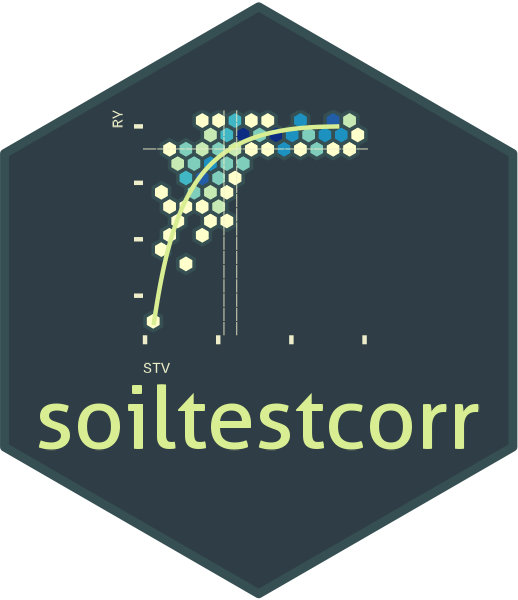## Description

This tutorial is intended to show how to deploy the quadratic_plateau() function for estimating a continuous response model with a critical soil test value. This function fits the classical regression response model that follows two phases: i) a first curvilinear phase described as y = b0 + b1 x + b2 x^2, and ii) a second phase were the RY response to increasing STV becomes NULL (flat), described as plateau = y = b0 + b1 Xc + b2 * Xc, where Xc represents the CSTV. The function works automatically with self starting initial values to facilitate the model convergence. This approach is a little more complex than linear-plateau, but the curvature of the response has more biological sense. Similar to linear-plateau, its disadvantages are that: i) the user does not have control to estimate the critical value (the model parameter) for an specific ry level; and ii) the confidence interval of the critical level is generally unreliable. We recommend the user to use a re-sampling technique (e.g. bootstrapping) for a more reliable confidence interval estimation for parameters and CSTV

## General Instructions

1. Load your dataframe with soil test value (stv) and relative yield (ry) data.

2. Specify the following arguments into the function -quadratic_plateau()-:

(a). data (optional),

(b). stv (soil test value) and ry (relative yield) columns or vectors,

(c). target (optional) if want a CSTV for a different ry than the plateau.

(d). tidy TRUE (produces a data.frame with results) or FALSE (store results as list),

(e). plot TRUE (produces a ggplot as main output) or FALSE (no plot, only results as data.frame),

(f). resid TRUE (produces plots with residuals analysis) or FALSE (no plot),

1. Run and check results.

2. Check residuals plot, and warnings related to potential limitations of this model.

3. Adjust curve plots as desired.

# Tutorial

library(soiltestcorr)

Suggested packages

# Install if needed
library(ggplot2) # Plots
library(dplyr) # Data wrangling
library(tidyr) # Data wrangling
library(utils) # Data wrangling
library(data.table) # Mapping
library(purrr) # Mapping

This is a basic example using three different datasets:


# Example 1 dataset
# Fake dataset manually created
data_1 <- data.frame("RY"  = c(65,80,85,88,90,94,93,96,97,95,98,100,99,99,100),
"STV" = c(1,2,3,4,5,6,7,8,9,10,11,12,13,14,15))

# Example 2. Native fake dataset from soiltestcorr package

data_2 <- soiltestcorr::data_test

# Example 3. Native dataset from soiltestcorr package, Freitas et al.  (1966), used by Cate & Nelson (1971)
data_3 <- soiltestcorr::freitas1966

## 1. Individual fits

RY target = 90%, confidence level = 0.95, replace with your desired values

### 1.1. tidy = FALSE

It returns a LIST (more efficient for multiple fits at once)


# Using dataframe argument, tidy = FALSE -> return a LIST
fit_1_tidy_false <-
ry = RY,
stv = STV,
tidy = FALSE)

#> $intercept #>  61.01 #> #>$slope
#>  8.59
#>
#> $equation #>  "61 + 8.59x + -0.5x^2 if x<CSTV" #> #>$plateau
#>  97.7
#>
#> $target #>  97.7 #> #>$CSTV
#>  8.6

### 1.2. tidy = TRUE

It returns a data.frame (more organized results)


# Using dataframe argument, tidy = FALSE -> return a LIST
fit_1_tidy_true <-
ry = RY,
stv = STV,
tidy = TRUE)

fit_1_tidy_true
#>   intercept slope                       equation plateau target CSTV LL_cxp
#> 1     61.01  8.59 61 + 8.59x + -0.5x^2 if x<CSTV    97.7   97.7  8.6    6.6
#>   UL_cxp AIC AICc   R2
#> 1   10.5  75   79 0.94

### 1.3. Alternative using the vectors

You can call stv and ry vectors using the $. The tidy argument still applies for controlling the output type  fit_1_vectors_list <- soiltestcorr::quadratic_plateau(ry = data_1$RY,
stv = data_1$STV, tidy = FALSE) fit_1_vectors_tidy <- soiltestcorr::quadratic_plateau(ry = data_1$RY,
stv = data_1$STV, tidy = TRUE) ### 1.4. Data 2. Test dataset  fit_2 <- soiltestcorr::quadratic_plateau(data = data_2, ry = RY, stv = STV) utils::head(fit_2) #>$intercept
#>  44.15
#>
#> $slope #>  2.86 #> #>$equation
#>  "44.1 + 2.86x + -0.04x^2 if x<CSTV"
#>
#> $plateau #>  96.4 #> #>$target
#>  96.4
#>
#> $CSTV #>  36.5 ### 1.5. Data 3. Freitas et al. 1966  fit_3 <- soiltestcorr::quadratic_plateau(data = data_3, ry = RY, stv = STK) utils::head(fit_3) #>$intercept
#>  12.86
#>
#> $slope #>  1.91 #> #>$equation
#>  "12.9 + 1.91x + -0.01x^2 if x<CSTV"
#>
#> $plateau #>  95.3 #> #>$target
#>  95.3
#>
stv = .$STV, tidy = TRUE))) utils::head(fit_multiple_map) #> # A tibble: 3 x 3 #> id data quadratic_plateau #> <chr> <list> <list> #> 1 1 <tibble [15 x 2]> <df [1 x 11]> #> 2 2 <tibble [137 x 2]> <df [1 x 11]> #> 3 3 <tibble [24 x 2]> <df [1 x 11]> ### 2.1. Using group_map Alternatively, with group_map, we do not require nested data. However, it requires to bind_rows and add an id column specifying the name of each dataset. This option return models as lists objects.  fit_multiple_group_map <- dplyr::bind_rows(data_1, data_2, .id = "id") %>% dplyr::group_by(id) %>% dplyr::group_map(~ soiltestcorr::quadratic_plateau(data = ., ry = RY, stv = STV, tidy = TRUE)) utils::head(fit_multiple_group_map) #> [] #> intercept slope equation plateau target CSTV LL_cxp #> 1 61.01 8.59 61 + 8.59x + -0.5x^2 if x<CSTV 97.7 97.7 8.6 6.6 #> UL_cxp AIC AICc R2 #> 1 10.5 75 79 0.94 #> #> [] #> intercept slope equation plateau target CSTV LL_cxp #> 1 44.15 2.86 44.1 + 2.86x + -0.04x^2 if x<CSTV 96.4 96.4 36.5 29.7 #> UL_cxp AIC AICc R2 #> 1 43.4 1023 1024 0.53 ## 3. Plots ### 3.1. Calibration Curve We can generate a ggplot with the same quadratic_plateau() function. We just need to specify the argument plot = TRUE.  quadratic_plateau_plot <- soiltestcorr::quadratic_plateau(data = data_3, ry = RY, stv = STK, plot = TRUE) quadratic_plateau_plot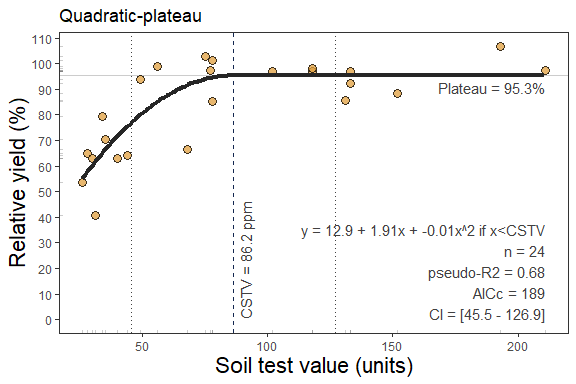### 3.1.2 Fine-tune the plots As ggplot object, plots can be adjusted in several ways. For example, modifying titles quadratic_plateau_plot_2 <- quadratic_plateau_plot + # Main title ggtitle("My own plot title")+ # Axis titles labs(x = "Soil Test K (ppm)", y = "Cotton RY(%)") quadratic_plateau_plot_2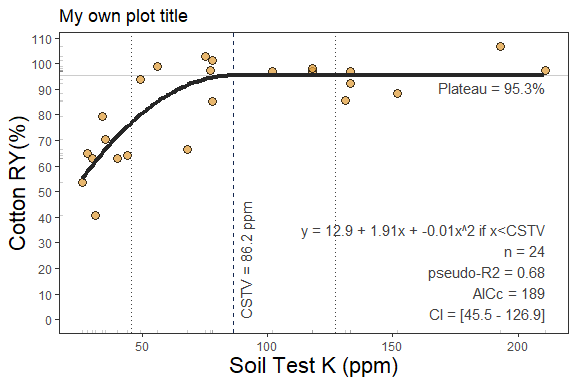Or modifying axis scales quadratic_plateau_plot_3 <- quadratic_plateau_plot_2 + # Axis scales scale_x_continuous(limits = c(20,220), breaks = seq(0,220, by = 20))+ # Axis limits scale_y_continuous(limits = c(30,100), breaks = seq(30,100, by = 10)) quadratic_plateau_plot_3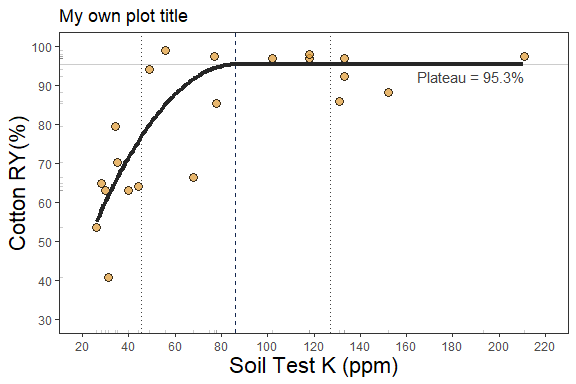### 3.3. Residuals We can generate a plot with the same quadratic_plateau() function. We just need to specify the argument resid = TRUE.  # Residuals plot soiltestcorr::quadratic_plateau(data = data_3, ry = RY, stv = STK, resid = TRUE)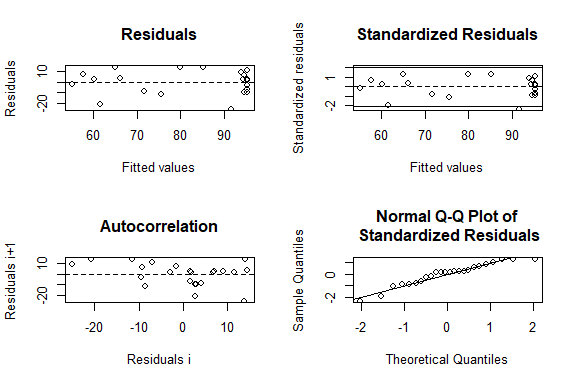#>$intercept
#>  12.86
#>
#> $slope #>  1.91 #> #>$equation
#>  "12.9 + 1.91x + -0.01x^2 if x<CSTV"
#>
#> $plateau #>  95.3 #> #>$target
#>  95.3
#>
#> $CSTV #>  86.2 #> #>$LL_cxp
#>  45.5
#>
#> $UL_cxp #>  126.9 #> #>$AIC
#>  187
#>
#> $AICc #>  189 #> #>$R2
#>  0.68

References

Bullock, D.G. and Bullock, D.S. (1994), Quadratic and Quadratic-Plus-Plateau Models for Predicting Optimal Nitrogen Rate of Corn: A Comparison. Agron. J., 86: 191-195. 10.2134/agronj1994.00021962008600010033x